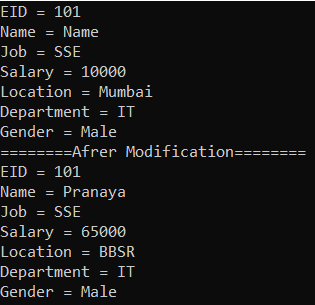# Indexers in C#

## Indexers in C# with Examples

In this article, I am going to discuss Indexers in C# with Examples. Please read our previous article where we discussed How to make Optional Parameters in C#. As part of this article, we will discuss what indexers are and how to create and use indexers in C#.

##### What are Indexers in C#?

The indexer in C# is a property of a class that allows us to access a member variable of a class using the features of an array. That means the Indexers in C# are the members of a class and if we define indexers in a class then the class behaves like a virtual array.

So, the Indexers in C# allow instances of a class to be indexed just like arrays. The indexed value can be set or retrieved without explicitly specifying a type or instance member. If this is not clear at the moment then don’t worry, we will understand this concept with examples.

##### Example to Understand Indexers in C#

Let us understand Indexers in C# with an example. Create a new console application with the name IndexersDemo. Then create a new class file with the name Employee.cs and then copy and paste the following code into it. The following class is very straightforward. We are just declaring some properties and initializing them through the class constructor.

```namespace IndexersDemo
{
public class Employee
{
//Declare the Properties
public int ID { get; set; }
public string Name { get; set; }
public string Job { get; set; }
public double Salary { get; set; }
public string Location { get; set; }
public string Department { get; set; }
public string Gender { get; set; }

//Initialize the Properties through Constructor
public Employee(int ID, string Name, string Job, int Salary, string Location,
string Department, string Gender)
{
this.ID = ID;
this.Name = Name;
this.Job = Job;
this.Salary = Salary;
this.Location = Location;
this.Department = Department;
this.Gender = Gender;
}
}
}```

Let’s try to create an instance of the above Employee class and let’s try to consume the employee object like an array. Let’s modify the Main method of the Program class as shown below. As you can see in the below code, first we create an instance of the Employee class and then we are trying to access the Employee data using index positions.

```using System;
namespace IndexersDemo
{
class Program
{
static void Main(string[] args)
{
//Creating the Employee instance
Employee emp = new Employee(101, "Pranaya", "SSE", 10000, "Mumbai", "IT", "Male");

//Accessing Employee Properties using Indexers i.e. using Index positions
Console.WriteLine("EID = " + emp);
Console.WriteLine("Name = " + emp);
Console.WriteLine("Job = " + emp);
Console.WriteLine("Salary = " + emp);
Console.WriteLine("Location = " + emp);
Console.WriteLine("Department = " + emp);
Console.WriteLine("Gender = " + emp);

}
}
}```

Now when you try to build the application, you will get the following error.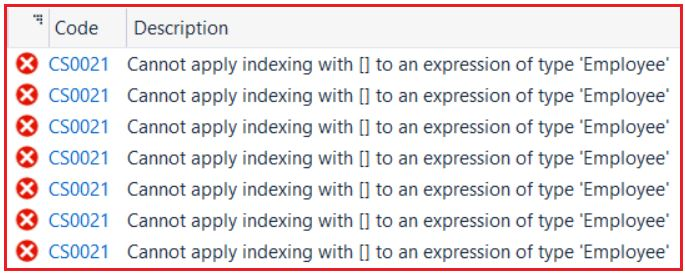This is because we cannot apply indexing directly to a class. We can do indexing on an array but we cannot do the same thing with a user-defined class like Employee. An array is a predefined class and all the logic is implemented in that class for indexing so that we can access them using indexes. But Employee is a user-defined class and we have not implemented any logic to access the class like an array.

If you want to access the class like an array, then first you need to define an indexer in the class. Once you define an indexer in the class, then only you can start accessing the values of the class by using the Indexer.

##### How to Define an Indexer in C#?

In C#, you need to use the following syntax to define an Indexer in an User-Defined Class.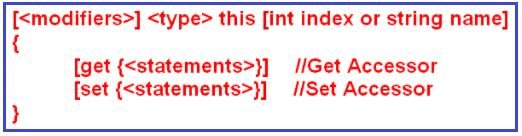Let us understand the above syntax:

1. Modifiers: The Modifiers are nothing but the access specifiers such as public, private, protected, internal, etc.
2. Type: In our class, as we are dealing with the integer (ID), string (Name, Job, Department, Location, and Gender), and double (Salary) type of values. So, here we need to use the type as an Object because the Object type can return any type of values.
3. This: This keyword tells that we are defining an indexer on the current class, in this case, the current class is Employee.
4. Int index or String name: The int index or string name is used to specify whether you want to access the values by using its integer index position or by using the string name.
5. Get and Set: The get accessor is used for returning the value and the set accessor is used for assigning the value.

Note: If the above syntax is not clear at the moment, then don’t worry, once we discussed the example, then you will definitely understand how to create and use Indexer in C#.

##### Example to Understand Indexers in C#:

Let us create an Indexer on the Employee class for both the get and set accessor. Please modify Employee.cs class file as shown below. Here, we create an Indexer by using the int index position so that we can access the elements by using its integer index position. Later  I will show you how to create Indexer using string name. In the case of the set accessor, the parameter “value” implicitly holds the assigned value.

```using System;
namespace IndexersDemo
{
public class Employee
{
//Declare the Properties
public int ID { get; set; }
public string Name { get; set; }
public string Job { get; set; }
public double Salary { get; set; }
public string Location { get; set; }
public string Department { get; set; }
public string Gender { get; set; }

//Initialize the Properties through Constructor
public Employee(int ID, string Name, string Job, int Salary, string Location,
string Department, string Gender)
{
this.ID = ID;
this.Name = Name;
this.Job = Job;
this.Salary = Salary;
this.Location = Location;
this.Department = Department;
this.Gender = Gender;
}

//Creating the Indexer using Integer Index Position
public object this[int index]
{
//Get accessor is used for returning a value
get
{
//based in the index position, return the appropriate property value
if (index == 0)
return ID;
else if (index == 1)
return Name;
else if (index == 2)
return Job;
else if (index == 3)
return Salary;
else if (index == 4)
return Location;
else if (index == 5)
return Department;
else if (index == 6)
return Gender;
else
return null;
}
//Set accessor is used to assigning a value
set
{
//based in the index position, set the appropriate property value
if (index == 0)
ID = Convert.ToInt32(value);
else if (index == 1)
Name = value.ToString();
else if (index == 2)
Job = value.ToString();
else if (index == 3)
Salary = Convert.ToDouble(value);
else if (index == 4)
Location = value.ToString();
else if (index == 5)
Department = value.ToString();
else if (index == 6)
Gender = value.ToString();
}
}
}
}```

Now, let’s try to access the values like an array, and also let’s try to modify the values like an array. So, please modify the Program class Main method as shown below.

```using System;
namespace IndexersDemo
{
class Program
{
static void Main(string[] args)
{
//Create an Instance of the Employee Class
Employee emp = new Employee(101, "Pranaya", "SSE", 10000, "Mumbai", "IT", "Male");

//Access the Employee Object using Indexer i.e. using Integer Index Position
Console.WriteLine("EID = " + emp);
Console.WriteLine("Name = " + emp);
Console.WriteLine("Job = " + emp);
Console.WriteLine("Salary = " + emp);
Console.WriteLine("Location = " + emp);
Console.WriteLine("Department = " + emp);
Console.WriteLine("Gender = " + emp);

//Set the Employee Object using Indexer i.e. using Integer Index Position
emp = "Kumar";
emp = 65000;
emp = "BBSR";

Console.WriteLine("========After Modification========");
//Access the Employee Object using Indexer i.e. using Integer Index Position
Console.WriteLine("EID = " + emp);
Console.WriteLine("Name = " + emp);
Console.WriteLine("Job = " + emp);
Console.WriteLine("Salary = " + emp);
Console.WriteLine("Location = " + emp);
Console.WriteLine("Department = " + emp);
Console.WriteLine("Gender = " + emp);

}
}
}```
###### Output: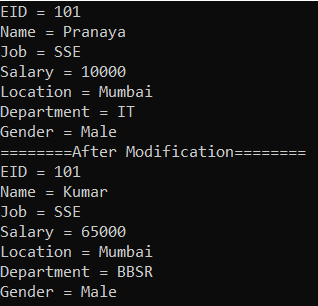##### Creating String Indexer in C#

In real-time, we may have more properties and it’s very difficult to access the values by using the integer index position. So in such cases, most of the time we need to access the values by using the property name. To do so we need to use a string name instead of an int indexer. So let’s modify the Employee class as shown below to use the string name as an indexer.

```using System;
namespace IndexersDemo
{
public class Employee
{
//Declare the Properties
public int ID { get; set; }
public string Name { get; set; }
public string Job { get; set; }
public double Salary { get; set; }
public string Location { get; set; }
public string Department { get; set; }
public string Gender { get; set; }

//Initialize the Propertities through constructor
public Employee(int ID, string Name, string Job, int Salary, string Location,
string Department, string Gender)
{
this.ID = ID;
this.Name = Name;
this.Job = Job;
this.Salary = Salary;
this.Location = Location;
this.Department = Department;
this.Gender = Gender;
}

//Creating the Indexer using string Index name
public object this[string Name]
{
//Get accessor is used for returning a value
get
{
//based in the index name, return the appropriate property value
if (Name == "ID")
return ID;
else if (Name == "Name")
return Name;
else if (Name == "Job")
return Job;
else if (Name == "Salary")
return Salary;
else if (Name == "Location")
return Location;
else if (Name == "Department")
return Department;
else if (Name == "Gender")
return Gender;
else
return null;
}
//Set accessor is used to assigning a value
set
{
//based in the index name, set the appropriate property value
if (Name == "ID")
ID = Convert.ToInt32(value);
else if (Name == "Name")
Name = value.ToString();
else if (Name == "Job")
Job = value.ToString();
else if (Name == "Salary")
Salary = Convert.ToDouble(value);
else if (Name == "Location")
Location = value.ToString();
else if (Name == "Department")
Department = value.ToString();
else if (Name == "Gender")
Gender = value.ToString();
}
}
}
}```

Next, modify the Main method of the Program class as shown below to use the String Indexer that we created inside the Employee class.

```using System;
namespace IndexersDemo
{
class Program
{
static void Main(string[] args)
{
//Create an Instance of the Employee Class
Employee emp = new Employee(101, "Pranaya", "SSE", 10000, "Mumbai", "IT", "Male");

//Access the Employee Object using Indexer i.e. using string Index name
Console.WriteLine("EID = " + emp["ID"]);
Console.WriteLine("Name = " + emp["Name"]);
Console.WriteLine("Job = " + emp["job"]);
Console.WriteLine("Salary = " + emp["salary"]);
Console.WriteLine("Location = " + emp["Location"]);
Console.WriteLine("Department = " + emp["department"]);
Console.WriteLine("Gender = " + emp["Gender"]);

//Set the Employee Object using Indexer i.e. using string Index name
emp["Name"] = "Kumar";
emp["salary"] = 65000;
emp["Location"] = "BBSR";

Console.WriteLine("========Afrer Modification========");

//Access the Employee Object using Indexer i.e. using string Index name
Console.WriteLine("EID = " + emp["ID"]);
Console.WriteLine("Name = " + emp["Name"]);
Console.WriteLine("Job = " + emp["job"]);
Console.WriteLine("Salary = " + emp["salary"]);
Console.WriteLine("Location = " + emp["Location"]);
Console.WriteLine("Department = " + emp["department"]);
Console.WriteLine("Gender = " + emp["Gender"]);

}
}
}```
###### Output: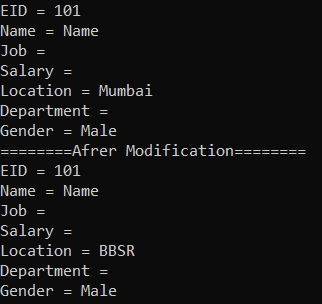As you can see, we are not getting the data for Job, Salary, and Department properties. This is because the Indexers in C# are case-sensitive. To get or set the data properly, either we need to convert the indexer name to upper or lower case or we need to use the string  Indexer name properly. To do so, modify the Employee class as shown below and here we are converting the Indexer name to Upper case.

```using System;
namespace IndexersDemo
{
public class Employee
{
//Declare the Properties
public int ID { get; set; }
public string Name { get; set; }
public string Job { get; set; }
public double Salary { get; set; }
public string Location { get; set; }
public string Department { get; set; }
public string Gender { get; set; }

//Initialize the Propertities through constructor
public Employee(int ID, string Name, string Job, int Salary, string Location,
string Department, string Gender)
{
this.ID = ID;
this.Name = Name;
this.Job = Job;
this.Salary = Salary;
this.Location = Location;
this.Department = Department;
this.Gender = Gender;
}

//Creating the Indexer using string Index name
public object this[string Name]
{
//Get accessor is used for returning a value
get
{
//based in the index name, return the appropriate property value
if (Name.ToUpper() == "ID")
return ID;
else if (Name.ToUpper() == "NAME")
return Name;
else if (Name.ToUpper() == "JOB")
return Job;
else if (Name.ToUpper() == "SALARY")
return Salary;
else if (Name.ToUpper() == "LOCATION")
return Location;
else if (Name.ToUpper() == "DEPARTMENT")
return Department;
else if (Name.ToUpper() == "GENDER")
return Gender;
else
return null;
}
//Set accessor is used to assigning a value
set
{
//based in the index name, set the appropriate property value
if (Name.ToUpper() == "ID")
ID = Convert.ToInt32(value);
else if (Name.ToUpper() == "NAME")
Name = value.ToString();
else if (Name.ToUpper() == "JOB")
Job = value.ToString();
else if (Name.ToUpper() == "SALARY")
Salary = Convert.ToDouble(value);
else if (Name.ToUpper() == "LOCATION")
Location = value.ToString();
else if (Name.ToUpper() == "DEPARTMENT")
Department = value.ToString();
else if (Name.ToUpper() == "GENDER")
Gender = value.ToString();
}
}
}
}```

Now, with the above changes in place, run the application and you will get the output as expected.

It is also possible in C# to overload Indexers in a Class. That means we can define multiple indexers in a class with the different data types for index. For a better understanding, please modify the Employee.cs class file as follows. As you can see in the below code, here we have two indexers with int type index as well as string type index.

```using System;
namespace IndexersDemo
{
public class Employee
{
//Declare the Properties
public int ID { get; set; }
public string Name { get; set; }
public string Job { get; set; }
public double Salary { get; set; }
public string Location { get; set; }
public string Department { get; set; }
public string Gender { get; set; }

//Initialize the Propertities through constructor
public Employee(int ID, string Name, string Job, int Salary, string Location,
string Department, string Gender)
{
this.ID = ID;
this.Name = Name;
this.Job = Job;
this.Salary = Salary;
this.Location = Location;
this.Department = Department;
this.Gender = Gender;
}

//Creating the Indexer using Integer Index Position
public object this[int index]
{
//Get accessor is used for returning a value
get
{
//based in the index position, return the appropriate property value
if (index == 0)
return ID;
else if (index == 1)
return Name;
else if (index == 2)
return Job;
else if (index == 3)
return Salary;
else if (index == 4)
return Location;
else if (index == 5)
return Department;
else if (index == 6)
return Gender;
else
return null;
}
//Set accessor is used to assigning a value
set
{
//based in the index position, set the appropriate property value
if (index == 0)
ID = Convert.ToInt32(value);
else if (index == 1)
Name = value.ToString();
else if (index == 2)
Job = value.ToString();
else if (index == 3)
Salary = Convert.ToDouble(value);
else if (index == 4)
Location = value.ToString();
else if (index == 5)
Department = value.ToString();
else if (index == 6)
Gender = value.ToString();
}
}

//Creating the Indexer using string Index name
public object this[string Name]
{
//Get accessor is used for returning a value
get
{
//based in the index name, return the appropriate property value
if (Name.ToUpper() == "ID")
return ID;
else if (Name.ToUpper() == "NAME")
return Name;
else if (Name.ToUpper() == "JOB")
return Job;
else if (Name.ToUpper() == "SALARY")
return Salary;
else if (Name.ToUpper() == "LOCATION")
return Location;
else if (Name.ToUpper() == "DEPARTMENT")
return Department;
else if (Name.ToUpper() == "GENDER")
return Gender;
else
return null;
}
//Set accessor is used to assigning a value
set
{
//based in the index name, set the appropriate property value
if (Name.ToUpper() == "ID")
ID = Convert.ToInt32(value);
else if (Name.ToUpper() == "NAME")
Name = value.ToString();
else if (Name.ToUpper() == "JOB")
Job = value.ToString();
else if (Name.ToUpper() == "SALARY")
Salary = Convert.ToDouble(value);
else if (Name.ToUpper() == "LOCATION")
Location = value.ToString();
else if (Name.ToUpper() == "DEPARTMENT")
Department = value.ToString();
else if (Name.ToUpper() == "GENDER")
Gender = value.ToString();
}
}
}
}```

Next modify the Main Method of the Program class as shown below. In the below code, we have created an instance of the Employee class and access the Employee object using both string and integer indexes.

```using System;
namespace IndexersDemo
{
class Program
{
static void Main(string[] args)
{
//Create an Instance of the Employee Class
Employee emp = new Employee(101, "Pranaya", "SSE", 10000, "Mumbai", "IT", "Male");

//Access the Employee Object using Indexer i.e. using string Index name
Console.WriteLine("EID = " + emp["ID"]);
Console.WriteLine("Name = " + emp["Name"]);
Console.WriteLine("Job = " + emp["job"]);
Console.WriteLine("Salary = " + emp["salary"]);
Console.WriteLine("Location = " + emp["Location"]);
Console.WriteLine("Department = " + emp["department"]);
Console.WriteLine("Gender = " + emp["Gender"]);

//Set the Employee Object using Indexer i.e. using string Index name
emp["Name"] = "Kumar";
emp["salary"] = 65000;
emp["Location"] = "BBSR";

Console.WriteLine("========Afrer Modification========");

//Access the Employee Object using Indexer i.e. using Integer Index Position
Console.WriteLine("EID = " + emp);
Console.WriteLine("Name = " + emp);
Console.WriteLine("Job = " + emp);
Console.WriteLine("Salary = " + emp);
Console.WriteLine("Location = " + emp);
Console.WriteLine("Department = " + emp);
Console.WriteLine("Gender = " + emp);